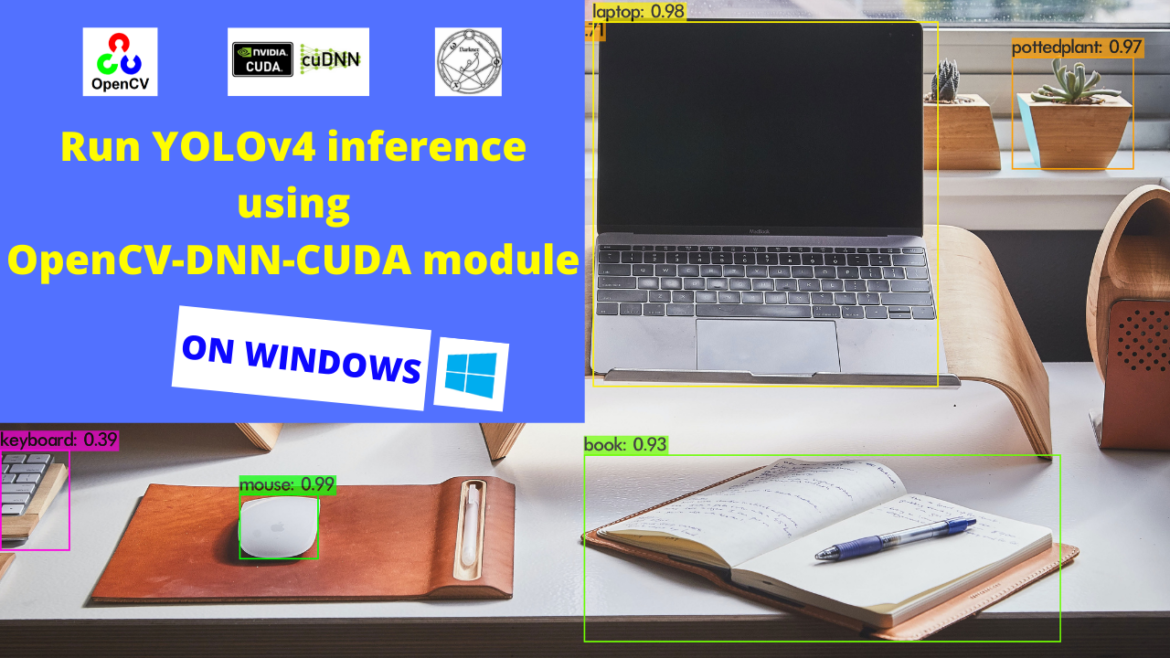# YOLOv4 inference using OpenCV-DNN-CUDA on Windows

### Run YOLOv4 inference using the OpenCV-DNN-CUDA module on Windows.

In the previous blog “Setup OpenCV-DNN module with CUDA backend support (For Windows)”, we built the OpenCV-DNN module with CUDA backend support on Windows. We can use this OpenCV module to run inference on object detection models. The OpenCV-DNN module built with CUDA backend support gives much faster inference as compared to the OpenCV without CUDA backend. All we need to do is add the following two lines to our code and inference the model using this.

``````net.setPreferableBackend(cv2.dnn.DNN_BACKEND_CUDA)
net.setPreferableTarget(cv2.dnn.DNN_TARGET_CUDA)``````

Follow the steps below to run inference using the OpenCV-DNN-CUDA module we have installed:

• Next, Download the following python opencv_dnn.py script and run it using python. Note that this uses the yolov4 model weights and config file. Download them from here.
```import cv2
import time

CONFIDENCE_THRESHOLD = 0.2
NMS_THRESHOLD = 0.4
COLORS = [(0, 255, 255), (255, 255, 0), (0, 255, 0), (255, 0, 0)]

class_names = []
with open("classes.txt", "r") as f:
class_names = [cname.strip() for cname in f.readlines()]

cap = cv2.VideoCapture("video.mp4")

net.setPreferableBackend(cv2.dnn.DNN_BACKEND_CUDA)
net.setPreferableTarget(cv2.dnn.DNN_TARGET_CUDA_FP16)

model = cv2.dnn_DetectionModel(net)
model.setInputParams(size=(416, 416), scale=1/255, swapRB=True)

while cv2.waitKey(1) < 1:
if not grabbed:
exit()

start = time.time()
classes, scores, boxes = model.detect(frame, CONFIDENCE_THRESHOLD, NMS_THRESHOLD)
end = time.time()

for (classid, score, box) in zip(classes, scores, boxes):
color = COLORS[int(classid) % len(COLORS)]
label = "%s : %f" % (class_names[classid], score)
cv2.rectangle(frame, box, color, 2)
cv2.putText(frame, label, (box, box-5), cv2.FONT_HERSHEY_SIMPLEX, 0.5, color, 2)

fps = "FPS: %.2f " % (1 / (end - start))
cv2.putText(frame, fps, (0, 25), cv2.FONT_HERSHEY_SIMPLEX, 1, (255, 0, 255), 2)
cv2.imshow("output", frame)
```